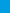4th Grade Common Core Math Workbook The Most Comprehensive Review for The Common Core State StandardsEffortless Math Common Core Workbook provides students with the confidence and math skills they need to succeed on the Common Core State Standards Math test, providing a solid foundation of basic Math topics with abundant exercises for each topic. It is designed to address the needs of students who must have a working knowledge of basic Math.\$10.99\$4.99
-55% only \$4.99

The Only Book Your student will Ever Need to ACE the Common Core Math Test!

Effortless Math Common Core Workbook provides students with the confidence and math skills they need to succeed on the Common Core State Standards Math test, providing a solid foundation of basic Math topics with abundant exercises for each topic. It is designed to address the needs of students who must have a working knowledge of basic Math.

This comprehensive workbook with over 2,500 sample questions and 2 complete 4th Grade Common Core Math tests is all a student needs to fully prepare for the Math tests. It will help students learn everything they need to ace the math exams.

There are more than 2,500 Math problems with answers in this book.

Effortless Math unique study program provides a student with an in-depth focus on the math test, helping them master the math skills that students find the most troublesome.

This workbook contains most common sample questions that are most likely to appear in the Common Core Math exams.

Inside the pages of this comprehensive workbook, students can learn basic math operations in a structured manner with a complete study program to help them understand essential math skills. It also has many exciting features, including:

• Dynamic design and easy-to-follow activities
• A fun, interactive and concrete learning process
• Targeted, skill-building practices
• Fun exercises that build confidence
• Math topics are grouped by category, so students can focus on the topics they struggle on
• All solutions for the exercises are included, so you will always find the answers
• 2 Complete Common Core Math Practice Tests

Effortless Common Core Math Workbook is an incredibly useful tool for those who want to review all topics being covered on the Common Core Math tests. It efficiently and effectively reinforces learning outcomes through engaging questions and repeated practice, helping students to quickly master basic Math skills.